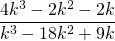Chapter 8: Rational Expressions

# 8.1 Reducing Rational Expressions

Definition: A rational expression can be defined as a simple fraction where either the numerator, denominator or both are polynomials. Care must be taken when solving rational expressions in that one must be careful of solutions yielding 0 in the denominator.

Rational expressions are expressions written as a quotient of polynomials. Examples of rational expressions include: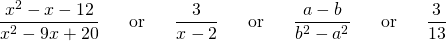As rational expressions are a special type of fraction, it is important to remember that you cannot have zero in the denominator of a fraction. For this reason, rational expressions have what are called excluded values that make the denominator equal zero if used.

Example 8.1.1

Find the excluded values for the following rational expression: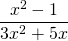For this expression, the excluded values are found by solving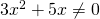.

Factor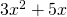first to yield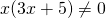.

There are now have two parts of this that can be made to equal 0: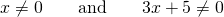Solving the second yields: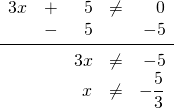As with our previous polynomials, evaluating rational expressions is easily accomplished by substituting the value for the variable and using order of operations.

Example 8.1.2

Evaluate the following rational expression for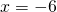: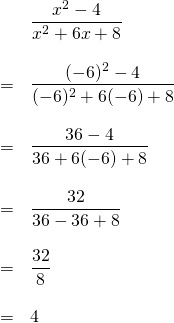Just as the expression was reduced in the previous example, often a rational expression can be reduced, even without knowing the value of the variable. When it is reduced, divide out common factors. This has already been seen with monomials when the properties of exponents was discussed. If the problem only has monomials, you can reduce the coefficients and subtract exponents on the variables.

Example 8.1.3

Reduce the following rational expression: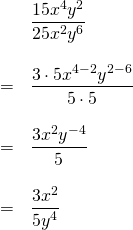If there is more than just one term in either the numerator or the denominator, you might need to first factor the numerator and denominator.

Example 8.1.4

Reduce the following rational expressions:

1.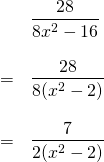2.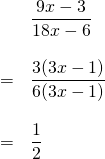3.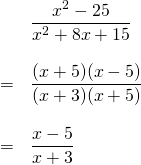# Questions

Evaluate Questions 1 to 6.

1.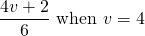2.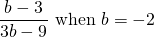3.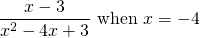4.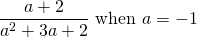5.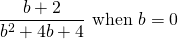6.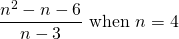For each of Questions 7 to 14, state the excluded values.

1.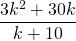2.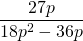3.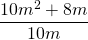4.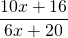5.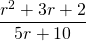6.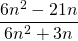7.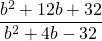8.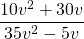For Questions 15 to 32, simplify each expression.

1.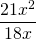2.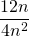3.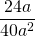4.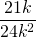5.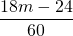6.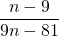7.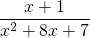8.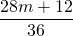9.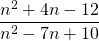10.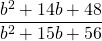11.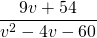12.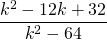13.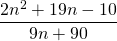14.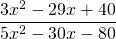15.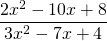16.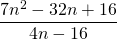17.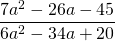18.